\$B:#>,\$N0lGU(B

\$BKhF|!":#>,\$N0lGU\$r>e\$2\$F\$\$\$^\$9!#(B
\$B:#>,0{\$`\$b\$N\$K:\$\$C\$?\$i!";29M\$K\$7\$F\$_\$F\$/\$@\$5\$\$!#(B

2023\$BG/(B6\$B7n(B

 1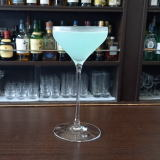(IM/D^3+0@0=(B 2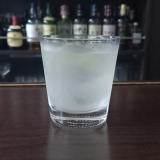(I;QW2%[/8(B 3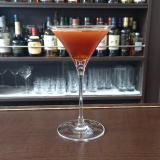(I7=%2]%;^%@^08(B 4 \$B5Y\$_(B 5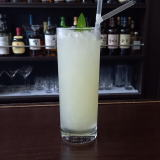(I80Y%:X]=^(B 6(IL*1X0%32=J_0(B 7 \$B5Y\$_(B 8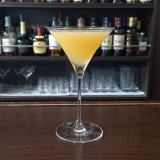(INIYY(B 9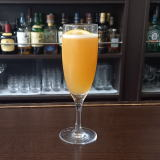(IL^W]C^0%;\0(B 10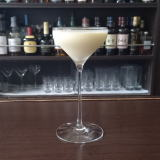(I:^0YC^]%D^X0Q(B 11 \$B5Y\$_(B 12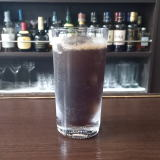(IK^J^%2@X1(B 13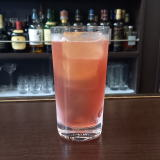(ILW]N^\0=^%?0@^(B 14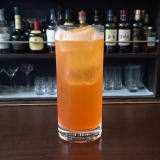(I1L_X:/D%80W0(B 15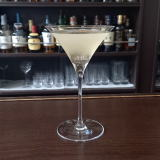(I=J_F/<-%@3](B 16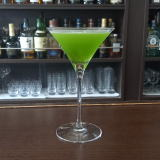(IC70W%<^,]8^Y(B 17(I1<^;2(B 18 \$B5Y\$_(B 19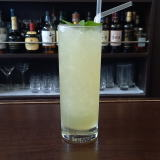(ISK0D(B 20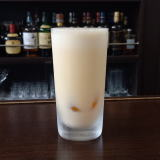(IPY8>07(B 21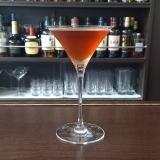(I<^](B&(I2/D(B 22(IN^Y90I(B 23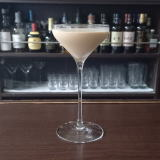(IW3^'0=^(B 24(IL(0Y%W28%NXC^0(B 25 \$B5Y\$_(B 26(I[]8^12W]D^%12=C(0(B 27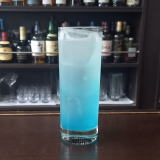(IL']@=C(/8%ZO](B 28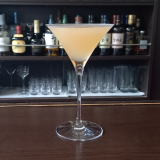(IXLZ/<-R]D(B 29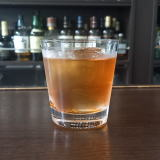(ILZ]A%:H8<.](B 30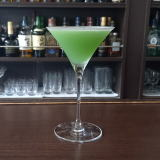(I8^W]%L^Y0(B